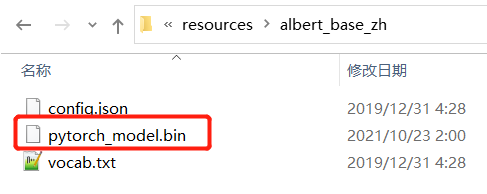• tensorflow版本的.ckpt模型转pytorch的.bin模型 - 最咸的鱼 - 博客园
将tensorflow版本的.ckpt模型转成pytorch的.bin模型 - 最咸的鱼 - 博客园
展开全文• 现在很多算法都是用pytorch框架训练的，但是在移动端部署很多又使用TensorFlow lite，...1、pytorch转onnx 这一步比较简单，使用pytorch自带接口就行。不过有一点需要注意的，就是opset版本，可能会影响后续的转换。
现在很多算法都是用pytorch框架训练的，但是在移动端部署很多又使用TensorFlow lite，因此需要将pytorch模型转换到TensorFlow lite。
将pytorch模型转到TensorFlow lite的流程是pytorch->onnx->tensorflow->tensorflow lite，本文记录一下踩坑的过程。
1、pytorch转onnx
这一步比较简单，使用pytorch自带接口就行。不过有一点需要注意的，就是opset版本，可能会影响后续的转换。
    os.environ['CUDA_VISIBLE_DEVICES']='0'
model_path = 'model.pth'
model = architecture.IMDN_RTC(upscale=2).cuda()

model.eval()
for k, v in model.named_parameters():

dummy_input = torch.rand(1, 3, 224, 224).cuda()
input_names = ["input"]
#output_names = ["output1", "output2", "output3"]
output_names = ["output"]
#使用pytorch的onnx模块来进行转换
#opset 10转换后，使用onnxruntime运行，在pixelshuffle处会出错
torch.onnx.export(model, dummy_input, "model.onnx", opset_version=11, verbose=True,
input_names=input_names, output_names=output_names,
dynamic_axes={'input': [0, 2, 3], 'output': [0, 2, 3]})

session = onnxruntime.InferenceSession("model.onnx")
input_name = session.get_inputs().name
#output_name = session.get_outputs().name
output_names = [s.name for s in session.get_outputs()]
input_shape = session.get_inputs().shape

img = np.transpose(img, (2, 0, 1)) / 255.
img = torch.from_numpy(img).unsqueeze(0).float()
res = session.run(output_names, {input_name: img.cpu().numpy()})
tmp = res
tmp = np.clip(tmp, 0, 1)
img = np.array(tmp*255, dtype=np.uint8)
img = np.transpose(img, (1, 2, 0))[:,:,::-1]
cv2.imwrite('tmp.jpg', img)
torch.onnx.export后，就得到了onnx模型，后面的代码是使用onnxruntime测试转换后的onnx模型。建议每一步转换后，都测试一下转换后模型的结果，确保每一步都是正确的。
2、onnx转TensorFlow
需要安装onnx-tensorflow进行转换。
from onnx_tf.backend import prepare
import onnx
import tensorflow as tf
if __name__ == '__main__':
tf_rep = prepare(onnx_model)  # prepare tf representation
tf_rep.export_graph("model.tf")  # export the model

img = np.transpose(img, (2, 0, 1)) / 255.
img = torch.from_numpy(img).unsqueeze(0).float()
input = img.numpy()
if 0:
output = tf_rep.run(input)  # run the loaded model
res = output.output
res = np.clip(res, 0, 1)
im = np.array(res*255, dtype=np.uint8)
im1 = np.transpose(im, (1, 2, 0))[:,:,::-1]
cv2.imwrite('tfres.jpg', im1)
else:
detect_fn = saved_model.signatures["serving_default"]
output = detect_fn(tf.constant(input))
tmp = np.array(output['output'])
res = np.clip(tmp, 0, 1)
im = np.array(res*255, dtype=np.uint8)
im1 = np.transpose(im, (1, 2, 0))[:,:,::-1]
cv2.imwrite('savedmodelres.jpg', im1)
转换部分就是前三行代码，后面是对TensorFlow模型的测试，确保转换结果没有问题。
3、TensorFlow转TensorFlow lite
没想到这一步是比较坑的，换了几个TensorFlow版本，最终使用tf2.5，转换成功了，参考issue。
import tensorflow as tf

if __name__ == '__main__':
# Convert the model
converter = tf.lite.TFLiteConverter.from_saved_model('model.tf') # path to the SavedModel directory
converter.target_spec.supported_ops = [
tf.lite.OpsSet.TFLITE_BUILTINS, # enable TensorFlow Lite ops.
tf.lite.OpsSet.SELECT_TF_OPS # enable TensorFlow ops.
]
tflite_model = converter.convert()

# Save the model.
with open('model.tflite', 'wb') as f:
f.write(tflite_model)

# test tflite model
interpreter = tf.lite.Interpreter(model_path='model.tflite')
#my_signature = interpreter.get_signature_runner()
img = np.transpose(img, (2, 0, 1)) / 255.
img = img[np.newaxis, :]
#output = my_signature(tf.constant(img))
print()

interpreter.resize_tensor_input(0, [1, 3, 256, 256])
interpreter.allocate_tensors()

# Get input and output tensors.
input_details = interpreter.get_input_details()
output_details = interpreter.get_output_details()

# Test the model on random input data.
#input_shape = input_details['shape_signature']
#input_data = np.array(np.random.random_sample(input_shape), dtype=np.float32)
input_data = img.astype(np.float32)
interpreter.set_tensor(input_details['index'], input_data)

interpreter.invoke()

# The function get_tensor() returns a copy of the tensor data.
# Use tensor() in order to get a pointer to the tensor.
output_data = interpreter.get_tensor(output_details['index'])
res = np.clip(output_data, 0, 1)
im = np.array(res*255, dtype=np.uint8)
im1 = np.transpose(im, (1, 2, 0))[:,:,::-1]
cv2.imwrite('tfliteres.jpg', im1)
这里需要注意一点，converter.target_spec.supported_ops这个需要加上，不然有些op在TensorFlow lite中不支持，转换不成功。


展开全文onnx 深度学习
• 由于目前 PyTorch 使用者非常多，BERT 源码又是 Google 用 TensorFlow 写的，谷歌...本文以 albert 模型为例，将 albert 的TensorFlow模型转PyTorch模型。 """ @Author : ChenXin @Data : 2021/10/23 1:26 @Brief : T
任务简介：
由于目前 PyTorch 使用者非常多，BERT 源码又是 Google 用 TensorFlow 写的，Google 开源的 BERT 预训练模型都是 TensorFlow 生成的 ckpt 模型文件， PyTorch 使用者需要将 ckpt 文件转换为 PyTorch 的 bin 模型文件。
本文以 albert 模型为例，将 albert 的 TensorFlow 模型转 PyTorch 模型。

albert 模型转换代码：
"""
@Author  : ChenXin
@Data    : 2021/10/23 1:26
@Brief   : TensorFlow 的模型转为 PyTorch 模型
"""
import argparse
import logging
import torch
from transformers import AlbertConfig, AlbertForPreTraining, load_tf_weights_in_albert

logging.basicConfig(level=logging.INFO)

def convert_tf_checkpoint_to_pytorch(tf_checkpoint_path, albert_config_file, pytorch_dump_path):
# 初始化 PyTorch 模型
config = AlbertConfig.from_json_file(albert_config_file)
print("Building PyTorch model from configuration: {}".format(str(config)))
model = AlbertForPreTraining(config)

# 从 tf 的 checkpoint 文件中加载模型权重

# 保存 PyTorch 模型
print("Save PyTorch model to {}".format(pytorch_dump_path))
torch.save(model.state_dict(), pytorch_dump_path)

if __name__ == "__main__":
parser = argparse.ArgumentParser()
# 参数设置
required=False, help="Path to the TensorFlow checkpoint path.")
required=False,
help="The config json file corresponding to the pre-trained ALBERT model. \n""This specifies the model architecture.", )
help="Path to the output PyTorch model.")

args = parser.parse_args()
convert_tf_checkpoint_to_pytorch(args.tf_checkpoint_path, args.albert_config_file, args.pytorch_dump_path)

输出：
生成了 PyTorch 模型展开全文深度学习
• 将这个阴影去除的tensorflow代码转pytorch。 3.过程 阅读需要复现的原文 很快啊，就一天可能就把TensorFlow的网络结构照猫画虎的写成了pytorch 然后就进入了无限调bug阶段。。。持续两周左右 最后想要放弃的时候，...
1. 前言
我想把一个TensorFlow代码转为pytorch代码，深度学习的代码。经过一个月的调试。。。。。自己好垃圾啊。。。
2.目标
将某tensorflow代码转pytorch。
3.过程
阅读需要复现的原文很快啊，就一天可能就把TensorFlow的网络结构照猫画虎的写成了pytorch然后就进入了无限调bug阶段。。。持续两周左右最后想要放弃的时候，打算搭建TensorFlow的环境跑作者提供的代码，这时灵光一现，发现了自己哪里写错了。。。
4.建议的过程
网络结构或许很简单，但是你如果不清楚细节，直接照猫画虎，那100%的出错，后期花费的时间将是超级大的代价！！！
4.1 论文阶段
粗度论文！粗读代码+精读论文。这一阶段一定要清晰的理解作者的思想，把代码和文章读通精读代码。这时把代码一字一字的读，我师兄说，他之前把别人的代码打印出来，一字一句的看，看到心中有数才开始写代码
4.2 代码阶段
TensorFlow的很多函数和Pytorch的函数、默认参数、初始化很多不一样，需要注意每个函数
5. 这一切的原因-分析
自己这段时间太急躁了！！！我可以静下心来看论文，但是一旦开始写代码，恨不得一天写完，写完了代码运行，感觉自己的干完了，但是写代码的过程中急躁是要不得的！！！写代码用了一天，调bug用了10天？事后回头看，总结一下自己的不足：
急躁不按部就班的做，一步一步的来，不要没学会走，就要跨栏。但是因为跨栏太过诱人，你非要跨栏？突然想起来飞蛾扑火这个词。代码复现不是科研！！是工程，所以要按照工程的思路来做，一步一步，走流程idea需要灵光一闪，但是写代码，一定要按部就班！！！不要偷懒，最开始想的装TensorFlow的环境太费劲，然后就复现pytorch的代码，所以就有了这糟心的一个月顶会的论文是有含金量的，在没有深入分析之后，切不可怀疑，复现就是复现！！！等你复现之后，在改动一些你认为可以提升性能的地方。就像英语翻译，老师一直教我们“信、达、雅”，保真是翻译的核心！！！
6.参数结构打印
TensorFlow1.12的打印结构：
for var in tf.trainable_variables():
print("Listing trainable variables ... ")
print(var)

TensorFlow1.12的打印参数：1
import tensorflow as tf

for key in global_variables:
print("tensor_name: ", key)

pytorch 打印结构：
net = resnet（）#实例化网络
print(net)

pytorch 打印参数：2
for name, parameters in net.named_parameters():#打印出每一层的参数的大小
print(name, ':', parameters.size())

for param_tensor in net.state_dict():  # 字典的遍历默认是遍历 key，所以param_tensor实际上是键值
print(param_tensor, '\t', net.state_dict()[param_tensor])

python打印到文本中：3
f = open('text.txt', 'w')
print('abc', file = f, flush=False)
f.close()


https://www.cnblogs.com/adong7639/p/7764769.html ↩︎ https://blog.csdn.net/chunfeng0301/article/details/108227660 ↩︎ https://www.zky.name/article/58.html ↩︎

展开全文• 最近做的一个事情需要将已经训练好的TF模型迁移到Pytorch上使用，于是在此记录一下。 一、方法一： 该方法比较“原始”，大致的做法是：1.读取TF模型-->2.获取参数名和值构成的字典-->3.构建Pytorch模型--&...python 深度学习
• 参考链接：... 一. tensorflow模型转pytorch模型 import tensorflow as tf import deepdish as dd import argparse import os import numpy as np def tr(v): # tensorflow weights to pytorch weights
• Transformers：支持TensorFlow 2.0 和 PyTorch 的自然语言处理预训练语言模型(BERT, GPT-2, RoBERTa, XLM, DistilBert, XLNet…) 8种架构/33种预训练模型/102种语言 用于 PyTorchTensorFlow 2.0 的最先进的自然...自然语言处理
• 关于如何将tensorflow模型转换为pytorch模型，https://www.cnblogs.com/cxq1126/p/14277134.html这个帖子讲的方法可以参考，不过会报错：AttributeError: ‘BertForPreTraining’ object has no attribute ‘shape’...
• 文章目录前世今生TensorFlow发展历程Google Jeff发布版本历程：2015年同类型框架有：发展2017年2019TensorFlow2.0 is coming编程风格对比 | 选择开启TensorFlow2.0为什么学习TensorFlowPytorch发展历程动态图Torch ...深度学习 人工智能
• Pytorch模型转Tensorflow模型部署上线pytorch模型tensorflow流程torch模型文件onnx文件.onnx文件tensorflow .pb文件导入计算图，测试样例注意版本差异和环境 最近在研究Query2Title模型，学术界上快速实验一般...深度学习 神经网络
• 您是否有一个使用TensorFlow的代码库和一个使用PyTorch的代码库，并且想要训练一个同时使用端到端的模型？ 该库无需重写任何一个代码库就可以实现！ 它允许您使用简单的函数包装TensorFlow图以使其可以通过...
• 1. pytorch转tensorflow # coding=utf-8 """Convert Huggingface Pytorch checkpoint to Tensorflow checkpoint.""" import argparse import os import numpy as np import tensorflow as tf import torch def...bert
• MMdnn是一个综合的跨框架工具，用于转换，可视化和诊断深度学习（DL）模型。 “ MM”代表模型管理，“ dnn”代表深度神经网络。 主要功能包括： 我们实现了一个通用转换器，可以在框架之间转换DL模型，这意味着您...
• 在本文中，我们将学习如何将PyTorch模型转换为TensorFlow。 如果您是深度学习的新手，那么使用哪种框架可能会让您不知所措。我们个人认为++PyTorch是您应该学习的第一个框架++，但它可能不是您想要学习的唯一框架。 ...
• 参考博客：https://towardsdatascience.com/converting-a-simple-deep-learning-model-from-pytorch-to-tensorflow-b6b353351f5d 未完待续...onnx
• TensorFlow相比，我很难掌握这个框架的核心概念。随后我把它放在我的“知识书架”上，接着就把它淡忘了。但是不久之前，PyTorch发布了一个新版本。所以，我决定再次给它一次机会。又过了一段时间，我终于明...
• ## pytorch模型转tensorflow pb模型

千次阅读 热门讨论 2020-04-24 17:11:21
#1.配置安装 conda create -n pth_pb python=3.7 pip install tensorflow==2.1.0 -i ...pip install tensorflow-addons==0.9.1 -i https://pypi.tuna.tsinghua.edu.cn/simp...深度学习
• 深度学习在过去十年获得了极大进展，出现很多新的模型，并且伴随TensorFlowPytorch框架的出现，有很多实现，但对于初学者和很多从业人员，如何选择合适的实现，是个选择。rasbt在Github上整理了关于深度学习模型...
• 使用pytorch_pretrained_bert将tensorflow模型转化为pytorch模型：https://blog.csdn.net/sunyueqinghit/article/details/103458365/ bert_config.json bert_model.ckpt.data-00000-of-00001 bert_model.ckpt.index ...checkpoint ckpt bert
• 近期任务，把论文中用Tensorflow实现的源码转换成Pytorch，菜鸡开始好好撸代码惹。。。 最开始进入Tensorflow中文社区和Pytorch中文社区过了一遍，发现没有特别能记住什么，还是看些基础的例子，然后动手实践起来，...
• 模型pytorch_model.bin成bert_model.ckpt的方法: import numpy as np import tensorflow as tf import torch v_list=[] for k,v in torch.load('pytorch_model.bin',map_location='cpu').items(): v_list....
• 1、安装pytorch 2、安装pytorch-transformers 3、下载模型，例如bert-base-chinese 4、转换 5、改名 【转换后的结果】 【修改目录名称、修改文件名称、可以删除ckpt文件】 这样就得到了转换好的模型 6...git
• ## TensorFlow与PyTorch

千次阅读 2020-10-31 16:46:08
TensorFlowPyTorch对比计算图分布式训练生产部署比较 参考链接： https://zhuanlan.zhihu.com/p/80733307 https://blog.csdn.net/qq_37388085/article/details/102559532 计算图 计算图是一种将计算描述成有向无环...python 深度学习
• AWS 是一组Docker映像，用于在TensorFlowTensorFlow 2，PyTorch和MXNet中训练和提供模型。 深度学习容器使用TensorFlow和MXNet，Nvidia CUDA（用于GPU实例）和英特尔MKL（用于CPU实例）库提供优化的环境，可在...
• 文章目录准备测试输入数据将Pytorch卷积层权重Tensorflow中将Pytorch DW卷积层权重Tensorflow中将Pytorch ...此时就会想些办法，将Pytorch官方提供的模型权重Tensorflow模型中。反之亦然。 首先，在Pyto深度学习 python...